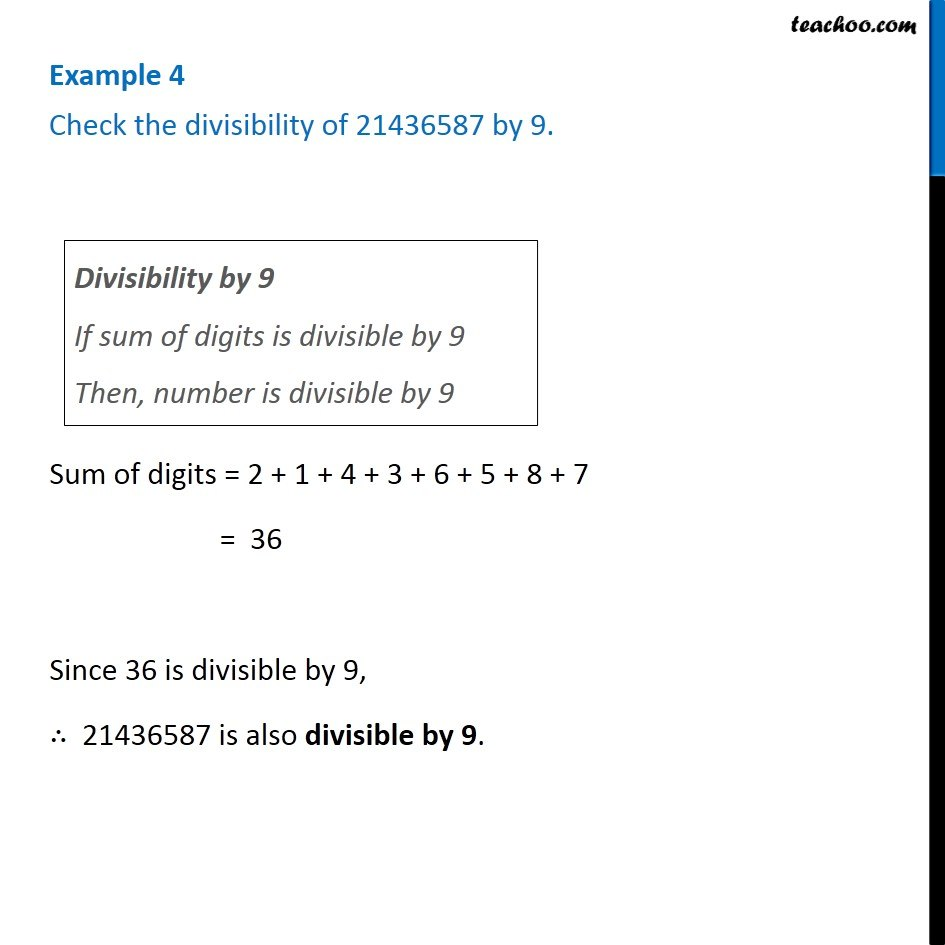Examples

Playing with Numbers
Serial order wiseLearn in your speed, with individual attention - Teachoo Maths 1-on-1 Class

### Transcript

Example 4 Check the divisibility of 21436587 byDivisibility by 9 If sum of digits is divisible by 9 Then, number is divisible by 9 9. Sum of digits = 2 + 1 + 4 + 3 + 6 + 5 + 8 + 7 = 36 Since 36 is divisible by 9, ∴ 21436587 is also divisible by 9.